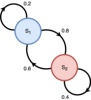Markov chain

1906年 

Andrey A. Markov

• 前言译自:《Training Restricted Boltzmann Machines: An Introduction 》 马尔科夫链在RBM的训练中占据重要地位，因为它提供了从复杂的概率分布(比如马尔科夫随机场MRF的吉布斯分布)中提取样本。这一部分主要就是对...
前言

译自:《Training Restricted Boltzmann Machines: An Introduction 》

马尔科夫链在RBM的训练中占据重要地位，因为它提供了从复杂的概率分布(比如马尔科夫随机场MRF的吉布斯分布)中提取样本。这一部分主要就是对马尔科夫链做个基本的理论介绍，将要着重强调的是，将吉布斯采样作为一种马尔科夫链蒙特卡洛方法去训练马尔科夫随机场以及训练RBM。

马尔科夫链

一个马尔科夫链是离散时间的随机过程，系统的下一个状态仅仅依赖当前的所处状态，与在它之前发生的事情无关。形式上，一个马尔科夫链是一组随机变量X={X(k)|k∈N0}$X=\{X^{(k)}|k\in N_0\}$，取值是一个有限集Ω$\Omega$，而且对于∀k≥0$\forall k\geq0$以及∀j,i,i0,⋯,ik−1∈Ω$\forall j,i,i_0,\cdots,i_{k-1}\in \Omega$都有
p(k)ij=Pr(X(k+1)=j|X(k)=i,X(k−1)=ik−1,⋯,X(0)=i0)=Pr(X(k+1)=j|X(k)=i)
\begin{aligned}
p_{ij}^{(k)}&=Pr(X^{(k+1)}=j|X^{(k)}=i,X^{(k-1)}=i_{k-1},\cdots,X^{(0)}=i_0)\\
&=Pr(X^{(k+1)}=j|X^{(k)}=i)
\end{aligned}

上式中表达出的‘无记忆’随机过程经常也被称为马尔科夫特性 ，如果对于所有k≥0$k\geq 0$的时间点，p(k)ij$p_{ij}^{(k)}$都有相同的pij$p_{ij}$(转移概率不会随着时间而改变)，这个链达到了稳态(homogeneous)，矩阵P=(pij)i,j∈Ω$P=(p_{ij})_{i,j\in \Omega}$称为稳态马尔科夫链的转移矩阵。

如果初始分布μ(0)$\mu^{(0)}$(即X(0)$X^{(0)}$的概率分布)是由概率向量μ(0)=(μ(0)(i))i∈Ω$\mu^{(0)}=(\mu^{(0)}(i))_{i\in\Omega}$给出的，其中μ(0)(i)=Pr(X(0)=i)$\mu^{(0)}(i)=Pr(X^{(0)}=i)$，那么X(k)$X^{(k)}$的分布μ(k)$\mu^{(k)}$是由μ(k)T=μ(0)TPk$\mu^{(k)T}=\mu^{(0)T}P^k$给出的。

对于πT=πTP$\pi^T=\pi^TP$中的π$\pi$，则称为稳态分布，如果马尔科夫链在k时刻达到稳态分布μ(k)=π$\mu^{(k)}=\pi$，那么所有的后续状态都是相同分布，也就是说对于所有的n∈N$n\in N$都有μ(k+n)=π$\mu^{(k+n)}=\pi$。关于马尔科夫链的分布π$\pi$为稳态分布的一个充分不必要条件是，对于转移概率pij,i,j∈Ω$p_{ij},i,j\in\Omega$中∀i,j∈Ω$\forall i,j\in\Omega$都有
π(i)pij=π(j)pji
\pi(i)p_{ij}=\pi(j)p_{ji}

这就称为细致平稳条件(detailed balanced condition)

对于马尔科夫链，存在唯一的一个稳态分布。这就是在有限状态空间Ω$\Omega$中，马尔科夫链不可约的案例。不可约的意思就是任何一个状态都能通过其它状态的有限次转移得到，公式表示就是，∀i,j∈Ω∃k>0$\forall i,j\in\Omega\quad\exists k >0$都有Pr(X(k)=j|X(0)=i)>0$Pr(X^{(k)}=j|X^{(0)}=i)>0$

如果链上所有的状态都是无规律发生的，就称为非周期性。公式表示就是，对于∀i∈Ω$\forall i\in \Omega$，集合k∈N0|Pr(X(k)=i|X(0)=i)>0$k\in N_0|Pr(X^{(k)}=i|X^{(0)}=i)>0$的所有元素的最大公约数是1。在有限状态空间中的，不可约，非周期性的马尔科夫链能够保证收敛到一个稳态分布。假设有限状态空间中有两个分布α$\alpha$和β$\beta$，变量距离可以被定义为
dV(α,β)=12|α−β|=12∑x∈Ω|α(x)−β(x)|
d_V(\alpha,\beta)=\frac{1}{2}|\alpha-\beta|=\frac{1}{2}\sum_{x\in\Omega}|\alpha(x)-\beta(x)|

为了方便标记，我们让行和列的概率向量作为上式的函数自变量，这样我们就有如下定理

假设π$\pi$是有限状态空间中的，不可约非周期的马尔科夫链的稳态分布，转移概率矩阵为P$\mathbf{P}$,对于任意的初始分布μ$\mu$都有
>limk→∞dV(μTPk,πT)=0>
> \lim_{k\to\infty}d_V(\mu^TP^k,\pi^T)=0
>

马尔科夫链蒙特卡洛方法，利用收敛定律，通过建立一个收敛到期望分布的马尔科夫链，然后从概率分布中生成样本。假设你想从具有有限状态空间的分布q中进行采样，随后就应该建立一个不可约、非周期的马尔科夫链，而且它的稳态分布π=q$\pi=q$。这是一个非平凡问题(non-trivial task)。如果k足够大，那么从马尔科夫链中重构X(k)$X^{(k)}$的状态x(k)$x^{(k)}$,就会逼近与π$\pi$中的一个样本,也是q$q$中的。吉布斯采样就是这样一种马尔科夫链蒙特卡洛MCMC方法。

吉布斯采样

吉布斯采样是一种简单的MCMC方法，从多元随机变量的联合概率分布中产生样本。最基本的想法就是，依据条件分布更新每一个变量，而条件分布的条件就是给定除此变量以外的其它变量的状态，如此构造一个马尔科夫链。随后我们将描述，如何从一个马尔科夫随机场MRF的吉布斯分布中，利用吉布斯采样生成(近似)样本。

我们假设一个马尔科夫随机场为X=(X1,⋯,XN)$X=(X_1,\cdots,X_N)$，即一个无向图模型G=(V,E)$G=(V,E)$，其中V={1,⋯,N}$V=\{1,\cdots,N\}$是为了做更清楚的标记。随机变量Xi,i∈V$X_i,i\in V$在有限集Λ$\Lambda$中取值，并且π(x)=1Ze−ε(x)$\pi(x)=\frac{1}{Z}e^{-\varepsilon (x)}$是X$X$的联合概率分布。此外，如果我们假设马尔科夫随机场随着时间改变状态，就可以将X={X(k)|k∈N0}$X=\{X^{(k)}|k\in N_0\}$当做从Ω=ΛN$\Omega=\Lambda^N$中取值的马尔科夫链。那么X(k)=(X(k)1,⋯,X(k)N)$X^{(k)}=(X_1^{(k)},\cdots,X_N^{(k)})$就描述了一个马尔科夫随机场在时刻k≥0$k\geq0$的状态。在接下来的两个后继时间节点中，链上新状态的产生都需要经过以下步骤

从概率q(i)$q(i)$中随机挑选一个变量Xi,i∈V$X_i,i\in V$，这里的概率q(i)$q(i)$是由V中的严格为正的概率分布q$q$给出的。
X(i)$X^{(i)}$的新状态就是给定其它所有变量(Xv)v∈V∖i$(X_v)_{v\in V\backslash i}$的状态(xv)v∖i$(x_v)_{v\backslash i}$，然后基于其条件概率分布采样得到的。依据条件随机场的局部马尔卡夫特性有π(xi|(xv)v∈V∖i)=π(xi|(xw)w∈ℵi)$\pi(x_i|(x_v)_v\in V\backslash i)=\pi(x_i|(x_w)_{w\in\aleph_i} )$。马尔科夫随机场的两个状态x,y,x≠y$x,y,x\neq y$的转移概率pxy$p_{xy}$是
pxy={q(i)π(yi|(xv)v∈V∖i),0,if ∃i∈Vso that ∀v∈Vwith v≠i:xv=yv)else
p_{xy}=\begin{cases}
\begin{aligned}
q(i)\pi(y_i|(x_v)_{v\in V\backslash i}),\quad&if \ \exists i\in V so\ that\  \forall v\in Vwith \ v\neq i:x_v=y_v)\\
\end{aligned}
\end{cases}

马尔科夫随机场x$x$的状态保持一致的概率，即pxx=∑i∈Vq(i)π(xi|(xv)v∈V∖i)$p_{xx}=\sum_{i\in V}q(i)\pi(x_i|(x_v)_{v\in V\backslash i})$

吉布斯链的收敛：为了证明由这些转移概率定义的马尔科夫链(因而被称作吉布斯连)，收敛到马尔科夫随机场的联合分布π$\pi$，我们需要证明π$\pi$是吉布斯链的稳态分布，而且这个链是不可约非周期的。

从细致平稳条件中，很容易发现π$\pi$是稳态分布：如果x$x$和y$y$在多个随机变量数值上有差异，就遵循一个事实pxy=Pyx=0$p_{xy}=P_{yx}=0$。假设x$x$和y$y$仅仅在一个确定的变量Xi$X_i$上的状态不同，比如当j≠i$j\neq i$的时候yj=xj$y_j=x_j$且yi≠xi$y_i\neq x_i$ ,那么
π(x)pxy=π(x)q(i)π(yi|(xv)v∈V∖i)=π(xi,(xv)v∈V∖i)q(i)π(yi,(xv)v∈V∖i)π((xv)v∈V∖i)=π(yi,(xv)v∈V∖i)q(i)π(xi,(xv)v∈V∖i)π((xv)v∈V∖i)=π(y)q(i)π(xi|(xv)v∈V∖i)=π(y)pyx
\begin{aligned}
\pi(x)p_{xy}&=\pi(x)q(i)\pi(y_i|(x_v)_{v\in V\backslash i})\\
&=\pi(x_i,(x_v)_{v\in V\backslash i})q(i)\frac{\pi(y_i,(x_v)_{v\in V\backslash i})}{\pi((x_v)_{v\in V\backslash i})}\\
&=\pi(y_i,(x_v)_{v\in V\backslash i})q(i)\frac{\pi(x_i,(x_v)_{v\in V\backslash i})}{\pi((x_v)_{v\in V\backslash i})}\\
&=\pi(y)q(i)\pi(x_i|(x_v)_{v\in V\backslash i})\\
&=\pi(y)p_{yx}
\end{aligned}

这样就满足了细致平稳条件，而且π$\pi$就是平稳分布。

因为π$\pi$是严格为正的，因而是单一变量的条件概率分布。这就意味着，每个单一变量Xi$X_i$在一个单一的转移步骤中，可以取每一个状态xi∈Λ$x_i\in \Lambda$，而且整个马尔科夫随机场中的每个状态都能经过有限步骤转移到ΛN$\Lambda^N$的任何其它状态。因此马尔科夫链就是不可约的。此外，对于所有的x∈ΛN$x\in \Lambda^N$，因为它还服从正的条件分布pxx>0$p_{xx}>0$，，所以这个马尔科夫链也是非周期的。不可约和非周期性就保证了链能够收敛到稳态分布π$\pi$

实际中，单个随机变量不是基于分布q$q$随机选择更新的，而是有一个固定的预定义顺序。对应的算法经常依赖于周期吉布斯采样器periodic Gibbs sampler。如果P$\mathbf{P}$是吉布斯链的转移矩阵，周期吉布斯采样器到马尔科夫随机场的稳态分布的收敛率，其界限可以使用下列不等式定义：
|μPk−π|≤12|μ−π|(1−e−NΔ)k
|\mu P^k-\pi|\leq\frac{1}{2}|\mu-\pi|(1-e^{-N\Delta})^k

其中Δ=supl∈Vδl$\Delta=\sup_{l\in V}\delta_l$，而且δl=sup{|ε(x)−ε(y)|;xi=yi ∀i∈V with i≠l}$\delta_l=\sup\{|\varepsilon(x)-\varepsilon(y)|;x_i=y_i\ \forall i\in V \ with\ i\neq l\}$,其中μ$\mu$是任意的起始分布，12|μ−π|$\frac{1}{2}|\mu-\pi|$是变量距离。这里有一个符号叫做sup$\sup$，表示数理统计中的格里文科(Gelivenko)定理

吉布斯采样和梅特罗波利斯哈斯廷斯算法 吉布斯采样属于梅特罗波利斯哈斯廷斯采样算法的一个更广泛的类别。这一类中所有的MCMC算法都利用两个步骤生成马尔科夫链的转移：

随机挑选一个候选状态，称为提议分布proposal distribution
候选状态根据一个接受概率acceptance probability，转移到马尔科夫链上的一个新状态，保证细致平稳条件

吉布斯采样的提议分布经常是翻转单个随机变量的当前状态，建议状态的接受概率就是一个条件概率，其条件就是给定的其它随机变量状态。

从易辛模型Ising Model中采样时，采样的提议分布(翻转状态)结合了接受概率 min(1,π(x′)π(x))$\min(1,\frac{\pi(x')}{\pi(x)})$，其中x$x$代表当前状态，x′$x'$代表马尔科夫链上的新状态。
展开全文机器学习 吉布斯采样
• 1马尔科夫链 在概率论里，弹硬币每次都是独立的，我上次弹是正面或反面完全对我下一次弹的结果没有任何影响。而世界上有很多事物前一次和后一次是有联系的，描述这种情况就可以利用马尔科夫链。 比如一台汽车的...
1马尔科夫链

在概率论里，弹硬币每次都是独立的，我上次弹是正面或反面完全对我下一次弹的结果没有任何影响。而世界上有很多事物前一次和后一次是有联系的，描述这种情况就可以利用马尔科夫链。

比如一台汽车的速度是连续变化的，它下一秒的速度肯定和上一秒有关系，并且是一个概率关系。比如一台跑车V=100时下一秒速度是120的概率是百分之50，但是一台卡车相同情况下下一秒是120的概率却很小，卡车和跑车的速度就是两个马尔科夫链模型。他是连续变化的。

所以马尔科夫链区别于独立抽样主要强调这个连续的关系。这里说的和前一秒有关系就是一阶的，和前2秒就是二阶的，以此类推。

2 隐马尔科夫链

参考http://blog.sina.com.cn/s/blog_953f8a550100zh35.html

隐马尔科夫链与马尔科夫链不同的就是，比如每天的温度是马尔科夫链，但是我观测不到温度，我没有温度计，我只能观测路人的穿多穿少情况，这肯定是随机的，因为再冷也有可能有人穿的少，但是比例会少。比如0度随便找个路人穿丝袜的概率肯定低，那我就以不同温度是否穿丝袜为准得到一组观测数据来推测一年里每天的温度.........我还真觉得每次统计全中国的比例还真有可能通过这个信息推算出一年里365天温度的变化。

展开全文• 马尔科夫链matlab程序包。马尔科夫链定义本身比较简单，它假设某一时刻状态转移的概率只依赖于它的前一个状态。举个形象的比喻，假如每天的天气是一个状态的话，那个今天是不是晴天只依赖于昨天的天气，而和前天的...
• 马尔科夫链蒙特卡洛方法的PPT很有用（含代码），很全面 有助于理解马尔科夫链蒙特卡洛方法MCMC(Markov Chain Monte Carlo)
• 概率论 马尔科夫链 排队 模拟 2017版本 英文原版教材；概率论 马尔科夫链 排队 模拟 2017版本 英文原版教材
• 马尔科夫链回顾 马尔科夫链是以俄国数学家安德烈⋅\cdot⋅马尔科夫命名，以纪念其首次提出马尔可夫链和对其收敛性质所做的研究。马尔科夫链是指数学中具有马尔科夫性质的离散事件随机过程。在给定当前知识或信息的...
马尔科夫链回顾
马尔科夫链是以俄国数学家安德烈$\cdot$马尔科夫命名，以纪念其首次提出马尔可夫链和对其收敛性质所做的研究。马尔科夫链是指数学中具有马尔科夫性质的离散事件随机过程。在给定当前知识或信息的情况下，过去（即当前以前的历史状态）对于预测将来（即当前以后的未来状态）是无关的，即过去与将来是独立的。
每个状态的转移只依赖于之前的$n$个状态，这个过程称为1个$n$阶的模型，其中$n$是影响转移状态的数目。最简单的马尔科夫过程就是一阶过程，每个状态的转移只依赖于之前的那一个状态。用数学表达式表示就是下面这个式子：$P(X_{n+1}=x|X_1=x_1,X_2=x_2,...,X_n=x_n)=P(X_{n+1}=x|X_n=x_n)$
用转移矩阵表示：$\mathtt{P^{(k)}}=\begin{bmatrix} p_{11}^k&p_{12}^k&\cdots&p_{1n}^k\\ p_{21}^k&p_{22}^k&\cdots&p_{2n}^k\\ \vdots&\vdots&\vdots&\vdots\\ p_{m1}^k&p_{m2}^k&\cdots&p_{mn}^k \end{bmatrix}$
其中$p_{ij}$就是根据上面那个公式计算出来的概率值。
$k=1$时为一步转移矩阵，当$k>1$时有$\mathtt{P^k}=\mathtt{P^{k-1}}\mathtt{P}$。经过多次迭代后，状态转移矩阵会趋于平衡，即当前时段和下一个时段的状态概率一定相同。
但是这并不是一个完美的模型。因为前后关系的缺失，导致信息的缺失。
比如我们的股市，如果只是观测市场，我们只知道当天的价格、成交量等信息，但是我们并不知道当前股市处于什么样的一个状态（牛市、熊市、震荡、反弹等等）。在这样的情况下，我们有两个集合，一个是可观测到的集合（股市价格成交量等信息），另一个是隐藏的状态集合（股市的状态）。
在这样的情况下，可以观察到的状态序列和隐藏的状态序列是概率相关的。于是我们可以将这种类型的过程建模为有一个隐藏的马尔科夫过程和一个与这个隐藏马尔科夫过程概率相关的并且可以观察到的状态集合，这就是隐马尔科夫模型。
隐马尔科夫链
下面给出隐马尔科夫链的定义。摘抄自李航的统计学习方法
隐马尔科夫模型是关于时序的概率模型，描述由一个隐藏的马尔科夫链随机生成不可观测的状态随机序列，再由各个状态生成一个观测而产生观测随机序列的过程。隐藏的马尔科夫链随机生成的状态的序列，称为状态序列；每个状态生成一个观测，而由此产生的观测的随机序列，称为观测序列。序列的每一个位置又可以看做是一个时刻。
隐马尔科夫模型由初始概率分布、状态转移概率分布以及观测概率分布确定。隐马尔科夫模型的形式定义如下：
设$Q$是所有可能的状态的集合，$V$是所有可能的观测的集合。$Q=｛q_1,q_2,...,q_N｝，V=｛v_1,v_2,...,v_M｝$其中，$N$是可能的状态数，$M$是可能的观测数。$I$是长度为$T$的状态序列，$O$是对应的观测序列。$I=｛i_1,i_2,...,i_T｝，O=｛o_1,o_2,...,o_T｝$$A$是状态转移概率矩阵：$A=[a_{ij}]_{N\times N}$其中，$a_{ij}=P(i_{t+1}=q_j|i_{t}=q_i),i,j=1,2,...,N$是在时刻$t$处于状态$q_i$的条件下在时刻$t+1$转移到状态$q_j$的概率。
$B$是观测概率矩阵：$B=[b_j(k)]_{N\times M}$其中，$b_j(k)=P(o_t=v_k|i_t=q_j), k=1,2,...,M;j=1,2,...,N$是时刻$t$处于状态$q_i$的条件下生成观测$v_k$的概率。
$\pi$是初始状态概率向量：$\pi=(\pi_i)$其中，$\pi_i=P(i_1=q_i),i=1,2,...,N$是时刻$t=1$处于状态$q_i$的概率。
隐马尔科夫模型由初始状态概率向量$\pi$、状态转移概率矩阵$A$以及观测概率矩阵$B$决定。$\pi$和$A$决定状态序列，$B$决定观测序列。因此，隐马尔科夫模型$\lambda$可用三元符号表示，即$\lambda=(A,B,\pi)$$A$、$B$、$\pi$称为隐马尔科夫模型的三要素。
状态转移概率矩阵$A$与初始状态概率向量$\pi$确定了隐藏的马尔科夫链，生成不可观测的状态序列。观测概率矩阵$B$确定了如何从状态生成观测，与状态序列综合确定了如何产生观测序列。
简单总结一下，隐马尔科夫模型是用来描述一个含有隐含未知参数的马尔科夫过程。其难点在于从可观察的参数中确定该过程的隐含参数。
简单例子
假设我手中有三个骰子，第一个骰子是常见的骰子（D6），6个面，每个面（1，2，3，4，5，6）出现的概率都是$\frac{1}{6}$；第二个骰子是个四面体（D4），4个面，每个面（1，2，3，4）出现的概率都是$\frac{1}{4}$；第三个骰子有八个面，每个面（1，2，3，4，5，6，7，8）出现的概率都是$\frac{1}{8}$。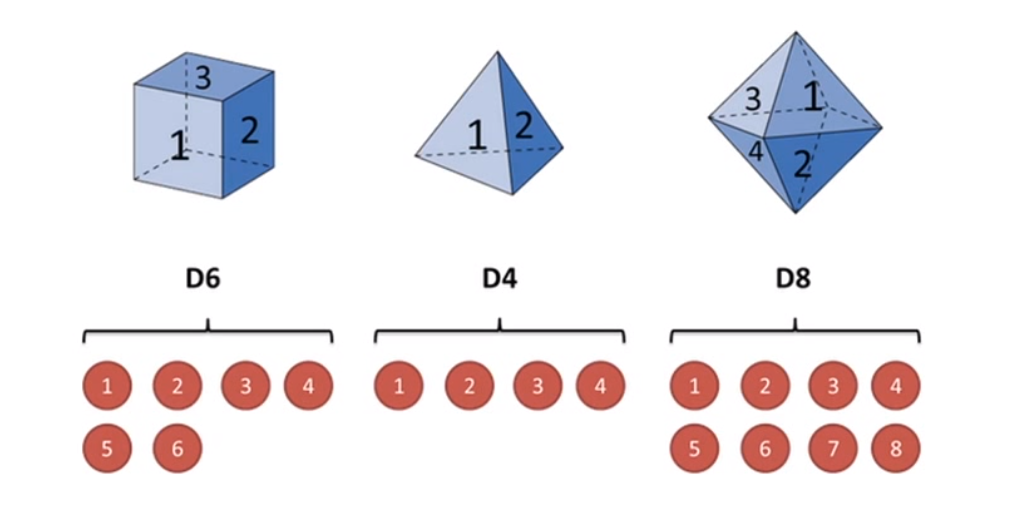假设我们开始掷骰子，我们先从D6、D4、D8之间选择一个，每一个骰子被挑到的概率都是$\frac{1}{3}$。然后我们掷骰子，得到一个数字，1，2，3，4，5，6，7，8中的一个。不停地重复，我们会得到一串数字，每个数字都是1~8中的一个。例如我们可能得到这么一串数字（掷骰子10次）：1，6，3，5，2，7，3，5，2，4。
这串数字叫做可见状态链。但是在隐马尔科夫模型中，我们不仅仅有这么一串可见状态链，还有一串隐含状态链。在这个例子里，隐含状态链就是用骰子的先后序列。比如可以是：D6，D8，D8，D6，D4，D8，D6，D6，D4，D8。
一般来讲，隐马尔科夫链中说到的马尔科夫链其实是指隐含状态链，因为隐含状态（骰子）之间存在转换概率。在这个例子里，D6下面一个状态是D6、D4、D8的概率都是$\frac{1}{3}$，同样对于D4和D8也是一样。
隐马尔科夫模型示意图：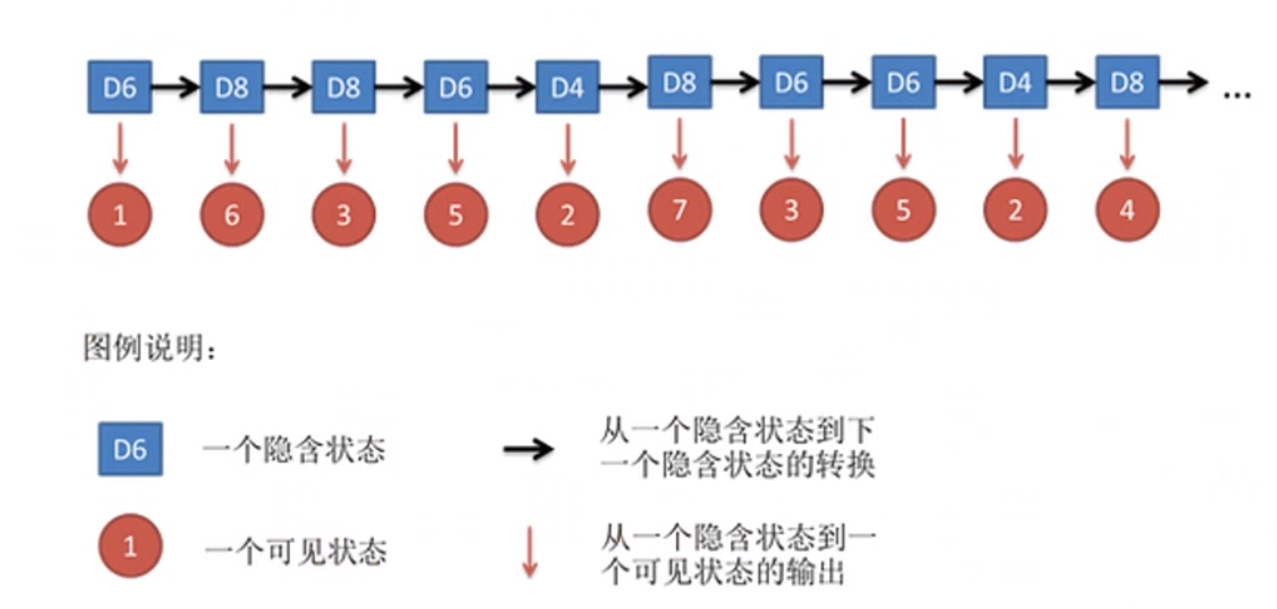隐含状态转换示意图：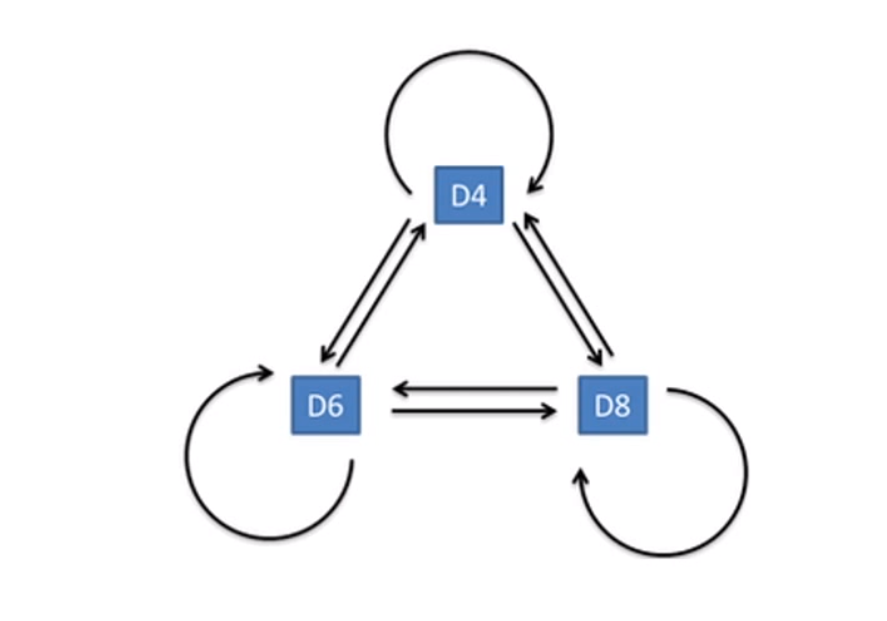我们其实可以随意设定转换概率。比方说，D6后面是D6的概率是0.9，是D8的概率是0.1，但是D6后面不能出现D4，所以D6后面是D4的概率是0。
同样的，尽管可见状态之间没有转换概率，但是隐含状态和可见状态之间有一个概率叫输出概率。比如说在我们这个例子，D6产生1的输出概率是$\frac{1}{6}$，同样产生2，3，4，5，6的输出概率也是$\frac{1}{6}$。我们同样可以人为定义输出概率，比如D6被人动过手脚，输出1的概率比较大是$\frac{1}{2}$，输出其他数字的概率是$\frac{1}{10}$。
我们可以模拟一下这个过程。假设$P$是D6、D4、D8之间转换的转换概率矩阵，$Q_i(i=6,4,8)$表示D6、D4、D8输出$(1,2,3,4,5,6,7,8)$的概率矩阵。现在有一个初始状态$\pi=[1,0,0]$（$\pi$的列标签是按照D6、D4、D8这个顺序来的），表示初始状态拿出的骰子是D6，则最终输出$(1,2,3,4,5,6,7,8)$的概率矩阵$X$应该等于：$X=\pi (PQ)(PQ)\cdots$这样就模拟出了整个掷骰子的过程。
但是在实际过程中，并不会这么简单，有可能不知道骰子的数目，或者这些骰子之间转换的概率，又或者这些骰子输出这些数字的概率是多少。比如在上面提到的股市，我们知道当天的价格成交量等信息，但是我们不知道当前股市处于什么样的一个状态。这就是一个典型的已知可见状态，未知隐含状态的问题。
隐马尔科夫链的三大问题
（1）已知观测序列$O=｛o_1,o_2,...,o_T｝$和模型$\lambda=(A,B,\pi)$，计算在该模型$\lambda$下观测序列$O$出现的概率$P(O|\lambda)$。
这是一个验证问题，就是说我们已经知道模型是什么样的，我们把观测数据给验证一下是否符合这个模型。
（2）已知观测序列$O=｛o_1,o_2,...,o_T｝$和模型$\lambda=(A,B,\pi)$，求给定观测序列条件概率$P(I|\lambda)$最大的状态序列$I=｛i_1,i_2,...,i_T｝$。即给定观测序列，求最有可能的对应的状态序列。
这是一个识别问题，我们从能观测的点识别出隐藏的状态，就是找到能够得到观测序列$O$的最优隐藏状态序列，即最优路径。
（3）已知观测序列$O=｛o_1,o_2,...,o_T｝$，估计模型$\lambda=(A,B,\pi)$的参数，使得在该模型下观测序列概率$P(O|\lambda)$最大。
这是一个训练学习的问题，就是说我们已经知道最终的结果是什么，但是我们不知道模型的参数，通过训练学习的方式，找到模型的参数最优解，使得观测序列概率$P(O|\lambda)$最大。
三大问题的英文描述：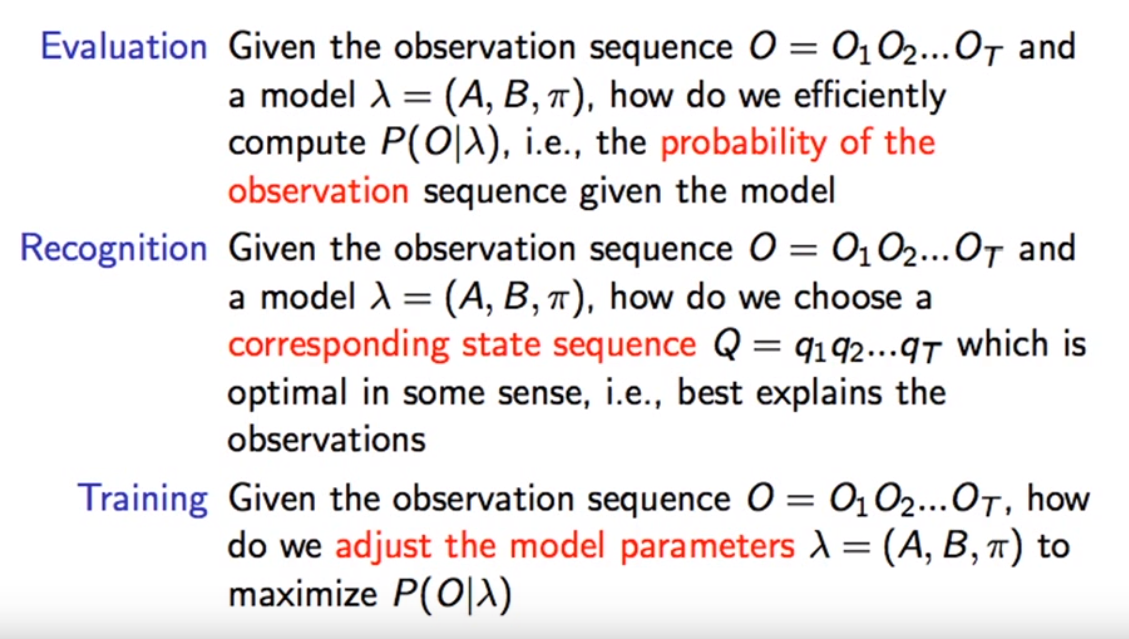关于解决这三大问题的方法，这里挖个坑，等之后有机会在回来填。


展开全文• 资料里包括了很多关于马尔科夫链的资料，有相关的PPT、Word以及代码等，适合初学者的学习...